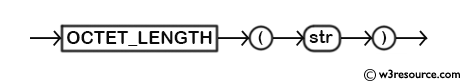# MySQL OCTET_LENGTH() function

## OCTET_LENGTH() function

MySQL OCTET_LENGTH() returns the length (in characters) of a string.

This function is useful in -

• Byte length calculation: It allows you to determine the length of a string in terms of the number of bytes it occupies.
• Data manipulation: Often, OCTET_LENGTH() is used to determine the length of strings for storage or transmission.

Syntax:

```OCTET_LENGTH(str)
```

Argument:

Name Description
str A string whose length is to be returned.

The above function is a synonym for LENGTH().

Syntax Diagram:MySQL Version: 8.0

Example: MySQL OCTET_LENGTH() function

The following MySQL statement will return the length of the string w3resource. The return value is 10.

Code:

``````SELECT OCTET_LENGTH("w3resource");
```
```

Output:

```mysql> SELECT OCTET_LENGTH("w3resource");
+----------------------------+
| OCTET_LENGTH("w3resource") |
+----------------------------+
|                         10 |
+----------------------------+
1 row in set (0.00 sec)
```

Video Presentation:

All String Functions (Slides presentation)

Previous: NOT REGEXP
Next: ORD

﻿